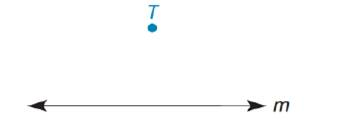Chapter 2.1, Problem 36E### Elementary Geometry for College St...

6th Edition
Daniel C. Alexander + 1 other
ISBN: 9781285195698

#### Solutions

Chapter
Section### Elementary Geometry for College St...

6th Edition
Daniel C. Alexander + 1 other
ISBN: 9781285195698
Textbook Problem
1 views

# Given: A line m and a point T not on mSuppose that you do the following:i) Construct a perpendicular line r from T to line m .ii) Construct a line s perpendicular to line r at point T .What is the relationship between lines s and m ?

To determine

To construct:

A perpendicular line r from T to line m and a line s perpendicular to line r at point T. Find the relationship between lines s and m.

Explanation

Approach:

Draw a straight line r from T in such a way that it is perpendicular to m.

Figure (1)

The line r from point T is perpendicular to line m is shown in figure (1).

Construct a straight line from point T in such a way that it is perpendicular to line r.

### Still sussing out bartleby?

Check out a sample textbook solution.

See a sample solution

#### The Solution to Your Study Problems

Bartleby provides explanations to thousands of textbook problems written by our experts, many with advanced degrees!

Get Started